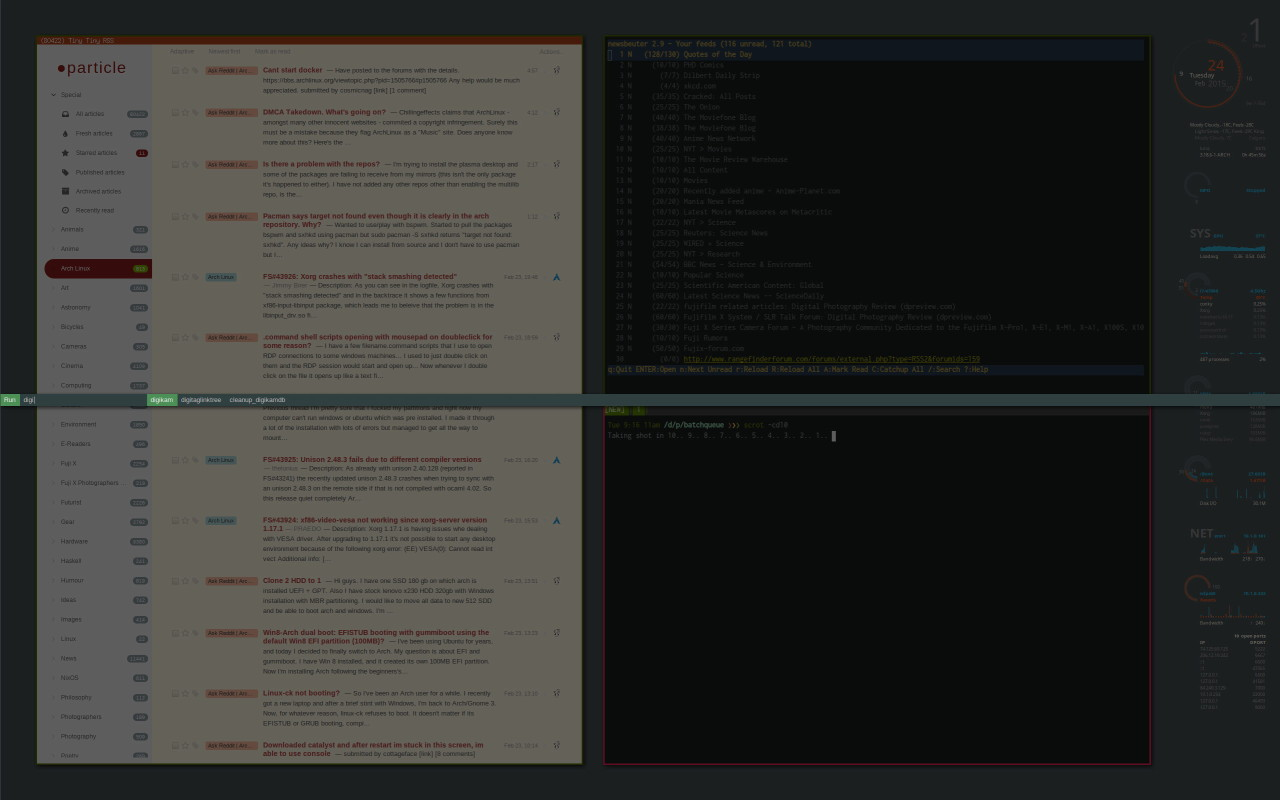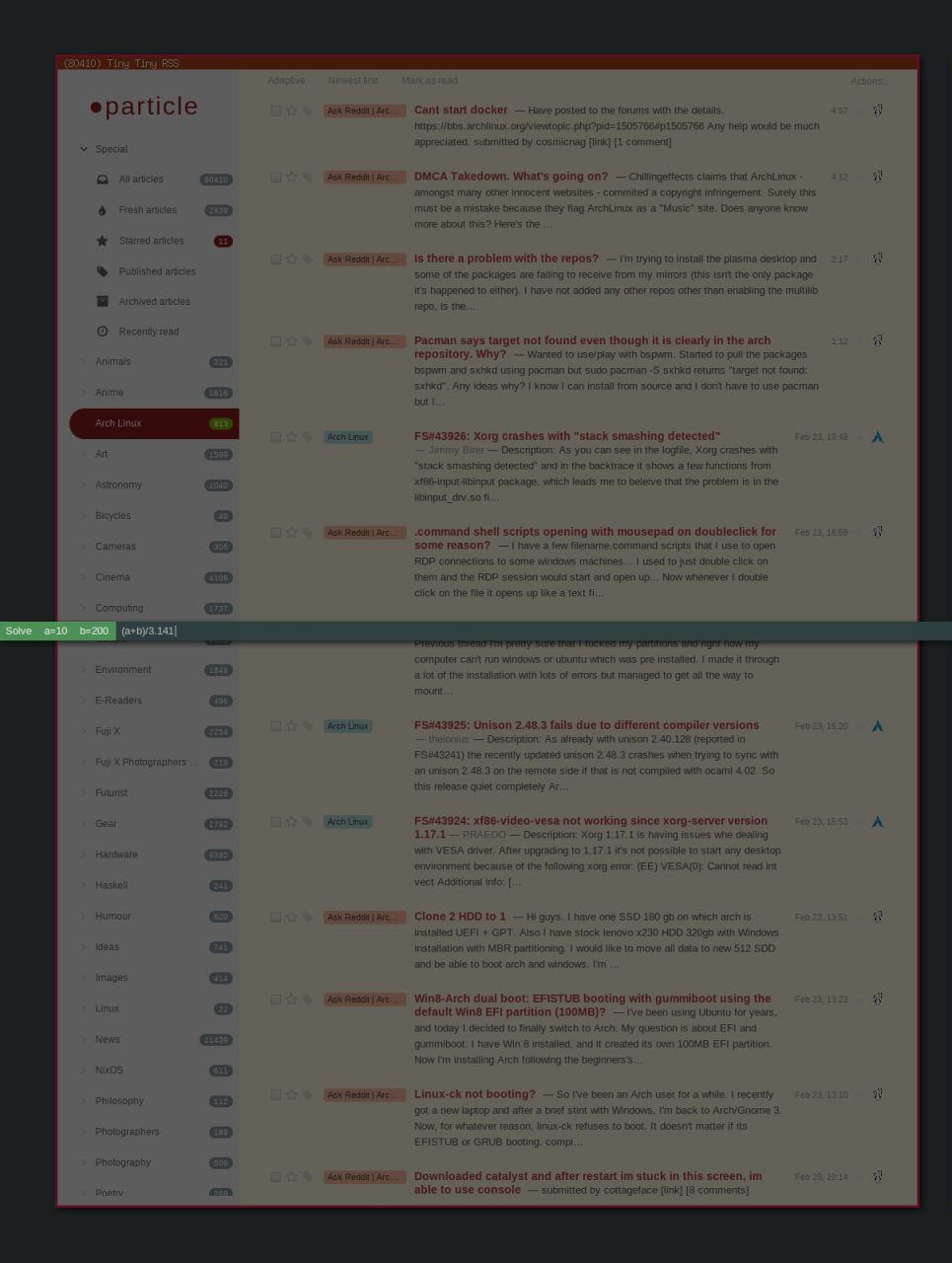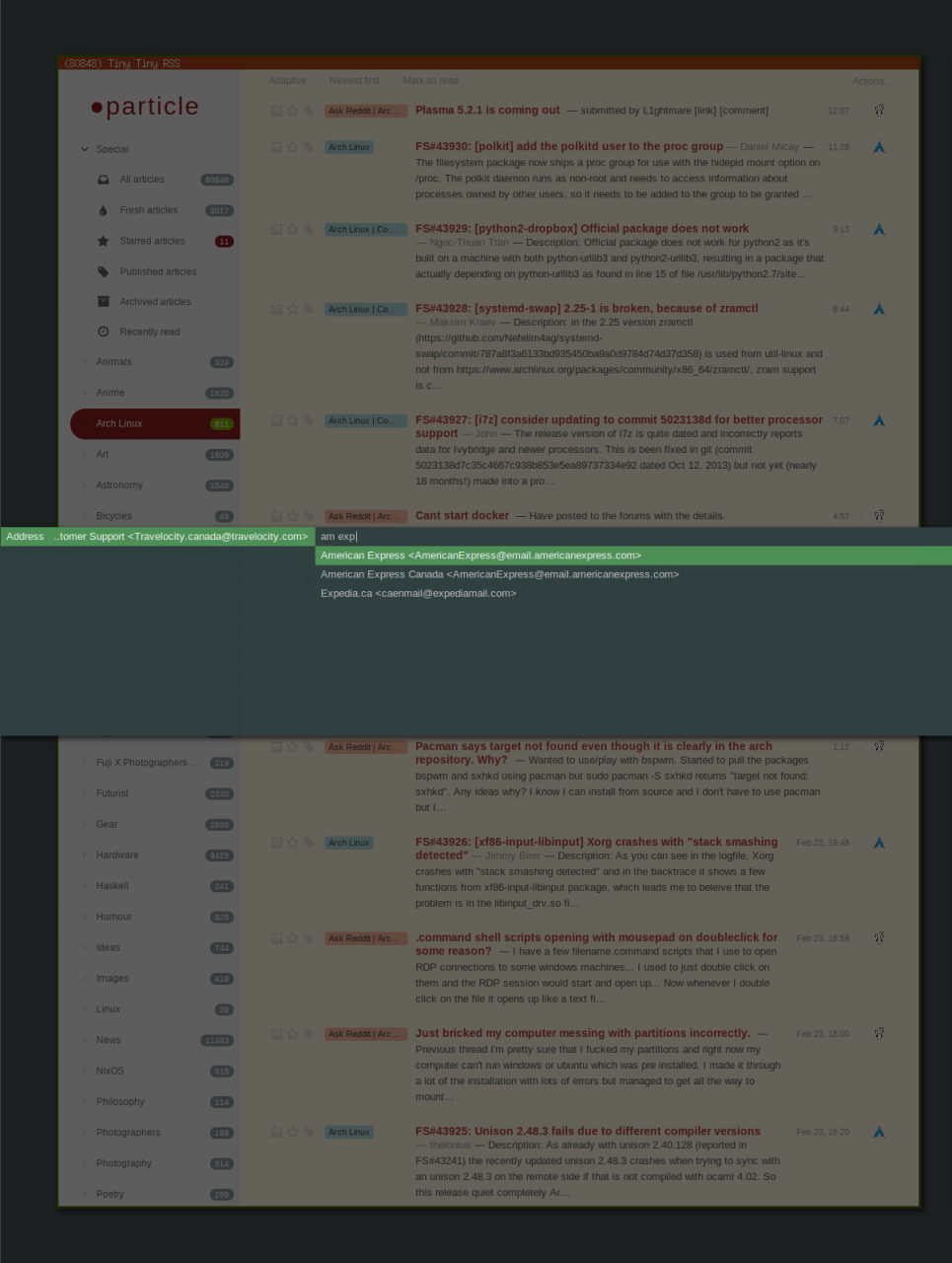wednesday, 25 february 2015

stumbling upon a extensively enhanced fork of a favourite applcation always raises my curiousity. dmenu2 doesn’t disappoint, including the available patches to dmenu plus adding several important UI enhancements.

Of particular interest to me are..

• vertical positioning of the dmenu prompt
• background dimming

The first without the latter would have required fine tuning the dmenu colours to obtain a suitable contrast with the applications in the background. But, with dimming—which requires a compositing manager— no changes to the existing dmenu themes are required, which now stand out much more clearly over the darkened background.It makes dmenu even better IMO..

## list mode

passes the list depth for..

`#!/bin/sh` `lines=10` `echo \$(dwrapper \$lines) -l \$lines`

centering the dmenu prompt..

`#!/bin/sh` `H=24` `[[ \$* ]] && height=\$(( \$H * (\$@ + 1) )) || height=\$H` `[[ \$(pidof compton) ]] && dim='-dim 0.6'` `theme=green` ` ` `function Y() {` ` case “\$@” in` ` left) Y=\$(( (1050 - \$height) / 2 )) ;;` ` right) Y=\$(( (1600 - \$height) / 2 )) ;;` ` laptop|*) Y=0 ;;` ` esac` `}` ` ` `Y=0` `case \$(cat ~/.windowmanager) in` ` herbstluftwm) if [[ \$(xdpyinfo | grep ‘dimensions:’ | grep -oE ‘[0-9]{1,}’ | sed -n 2p) -gt 600 ]]; then` ` focus=\$(herbstclient list_monitors | grep ‘[FOCUS]’ | cut -d: -f1)` ` case \$focus in` ` 0) Y ‘right’ ;;` ` 1) Y ‘left’ ;;` ` esac` ` fi` ` ;;` ` bspwm) focus=\$(bspc query –monitors –monitor focused)` ` case \$focus in` ` DVI-0) Y ‘left’ ;;` ` DVI-1) Y ‘right’ ;;` ` esac` ` ;;` `esac` ` ` `function dmenu() {` ` echo /usr/bin/dmenu -i \$dim -y \$Y -h \$H -fn “Arial-10” -nb “#333” \$@` `}` ` ` `case \$theme in` ` red) dmenu -sb “#d00” -sf “#fff” ;;` ` darkred) dmenu -sb “#b5423f” ;;` ` yellow) dmenu -sb “#ffde1e” -sf “#333” ;;` ` darkyellow) dmenu -sb “#fd0” -sf “#555” ;;` ` green) dmenu -sb “#4E9258” -sf “#fff” ;;` ` darkgreen) dmenu -sb “#080” -sf “#fff” ;;` ` blue) dmenu -sb “#099” ;;` ` darkblue|*) dmenu ;;` `esac`

The screen height is specific to my multihead setup. Dim is only set if the compton compositing manager is running—else the background would be blanked out.

## ribbon calculator

is enhanced to store and list each computed result in a variable a..z in the dmenu prompt which can be referenced (by variable name) in subsequent calculations..

`#!/bin/sh` `vars=( \$(echo {a..z}) )` `for i in {a..z}` `do` ` eval \$i=..` `done` `count=-1` `ribbon='Solve'` ` ` `function eqn() {` ` echo \$eqn | sed -e ‘s/([a-z])/\$\1/g’ ` ` -e ‘s/([()])/\\1/g’ ` ` -e ‘s/([<>])/../g’ ` ` -e ‘s/[\$](.)[\$]/\$\1..\$/g’` `}` ` ` `while eqn=\$(\$(dwrapper) -noinput -p "\$ribbon\$error")` `do` ` [[ \$eqn ]] || break` ` if ans=\$(calc -pd “\$(eval echo \$(eqn))”); then` ` if [[ \$ans ]]; then` ` if echo \$ans | grep -v ‘Error|Warning’; then` ` echo -n “\$ans” | xsel -i` ` count=\$(( \$count + 1 ))` ` [[ \$count -gt 25 ]] && count=0` ` eval \${vars[\$count]}=\$ans` ` ribbon=”\$ribbon \$(eval echo \${vars[\$count]}=\$ans)”` ` unset error` ` else` ` unset ans` ` fi` ` fi` ` else` ` unset ans` ` fi` ` [[ \$ans ]] || error=” ?=\$eqn”` `done` ` ` `if [[ \$ans ]]; then` ` echo -n “\$ans” | xsel -i` ` time=10000 notify “Ctrl-Alt-V” “\$ans”` `fi`

The -noinput option suppresses highlighting an empty field produced by the conventional “echo | dmenu..” usage—a very minor visual cleanup.`...` `function ribbon() {` ` width=50` ` if [[ -z \$* ]]; then` ` echo` ` elif [[ \$(echo \$@ | wc -c) -lt \$width ]]; then` ` echo “ \$@”` ` else` ` echo “ ..\$(echo \$@ | cut -c \$(( \$(echo \$@ | wc -c) - \$width ))-)”` ` fi` `}` ` ` `while true` `do` ` address=\$(cat \$addressbook | sed -e ‘1i[ clipboard ]\n[ rebuild database ]’ | \$(dlist) -p “Address\$(ribbon \$addresses)”) || break` ` case “\$address” in` ` ‘[ clipboard ]’) break ;;` ` ‘[ rebuild database ]’) dinit ;;` ` ) address=\$(echo \$address | sed ‘s/. :: //’)` ` [[ \$addresses ]] && addresses=\$addresses,\$address || addresses=\$address ;;` ` esac` `done` `...`